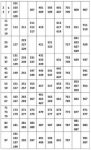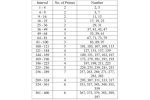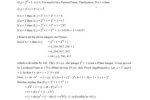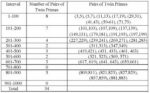# Learning Maths | Perfect and prime numbers

#### Perfect Number is one of the most fascinating and interesting numbers

Perfect Number: Among the different types of numbers we learn in Mathematics, Perfect Number is one of the most fascinating and interesting numbers. If the sum of all positive divisors or factors excluding the number itself is equal to the given number, then this number is called Perfect Number. Sometimes it is called an ideal or a complete number. Mathematically, a positive integer ‘n’ is said to be a Perfect number if o(n) = 2n where ‘o’ is small sigma.

Example: Positive divisors of 6 (excluding the number itself) are 1, 2 and 3, sum of all the positive divisors (1+2+3) = 6, which is equal to the number itself. Therefore, ‘6’ is the smallest perfect number.

In about 300 BC, Greek Mathematician Euclid showed in the Elements Book IV that if (2m-1) is a prime number, then (2m-1) 2m-1 is a perfect one. The first four Perfect numbers i.e. 6, 28, 496 and 8128 were the only ones known to early Greek Mathematics and the mathematician Nicomachus (introductio Arithmeticae) had noted 8128 as early as 100 AD. Only these four Perfect numbers are less than 10,000. But the fifth Perfect No. 33,550,336=212 (213-1) was invented by the Egyptian mathematician Ismail ibn fallus (1194 – 1252) nearly 1700 years after the last invention. Ismail mentioned the next two perfect numbers 8,589,869,056 = [216 (217 – 1)] and 137, 438, 691, 328.

In 1588, Italian Mathematician Pietro Cataldi also identified the sixth (8, 589, 869, 056) and seventh (137, 438, 691, 328) Perfect numbers and also proved that every perfect number obtained from Euclid’s rule ends with a 6 or an 8. Perhaps you have noticed that all perfect numbers are even numbers.

The first four perfect numbers are generated by the formula 2m- 1 (2m-1) with m is a prime number as follows:

If m = 2 then 21 (22 – 1)=6
If m = 3 then 22 (23 – 1)=28
If m = 4 then 24 (25 – 1)=496
If m = 7 then 26 (27 – 1)=8128

Nicomachus (60-120 AD) conjectured that every perfect number is of the form 2m – 1 (2m – 1) where (2m – 1) is prime.

In the 18th Century, Leonhard Euler proved that the formula 2m – 1 (2m-1) will produce all the even perfect numbers. Thus, there is a one-to-one correspondence between even perfect number and Mersenne primes, each Mersenne Prime generates one even perfect number, and vice versa. Remember that prime numbers of the form (2m-1), m is a Prime No are known as Mersenne Primes, after the 17th Century Monk Marin Mersenne introduced this formula, he studied the number theory and perfect numbers as well as having the form 2m1 (2m-1), each even perfect number is the (2m1)th triangular number (and hence equal to the sum of the integers from 1 to 2m – 1) and the 2m- 1th hexagonal number.

Furthermore, each even perfect number except for 6 is the (2m + 1)/3 th Centered nonagonal number and is equal to the sum of the first 2 odd cubes.

It is an unanswered question whether there is any odd perfect number, though various results have been obtained. In 1496, Jacques Lefevre stated that Euclid’s rule gives all perfect numbers, thus implying that no odd perfect exists. More recently, Carl Pomerance has presented a heuristic argument suggesting that indeed no odd perfect number should exist.

A pair of numbers is amicable if each is the sum of the proper divisors of the others.

The smallest pair is 220 and 284. The prime factorisation of 220 = 22 x 5 x 11

Sum of the proper divisors = 1+2+4+5+10+11+20+22+44+55 +110=284

And Similarly 284=22×71 and sum of its proper divisors = 1+2+4+71+142=220

These numbers have a particular influence in establishing union and friendship between individuals. Thabit ibn Qurra (C. AD 850) in his book on the, determination of Amicable Numbers noted that if you choose so that each of the expression a = 3.2P-1, b = 3.2P-1 – 1 and c = 9.22P-1 – 1 is Prime then 2Pab and 2PC are amicable Numbers. The 2nd pair, 17,296 and 18,416 was discovered by Ibn al – Banna (1256 – 1321) and redis covered by Peirre De Fermat in 1636. Decartes found the third pair 9,363,584 and 9,437.056 using Thabit’s formulae in this case P = 7. Now another type of amicable pair is paganini amicable pair, 1184 and 1210, is named after Nicolo Paganini, who discovered them in 1866. More than 7,500 amicable pairs have been found using computers.

## PRIME NUMBERS

After the death of Euclid, Alexandrian Mathematician Eratosthenes of Cyrene (276 – 194 BC) worked in number theory. He was known as 2nd Plato. He gave first the concept of Prime numbers. The word ‘Prime’ has derived from old English ‘Prim’ which comes from Latin ‘Prima hora’ means first hour. A positive number which is divisible only by I and itself is called a Prime number, the integers 2, 3, 5, 7, 11, 13 …… 113 etc are example of Prime numbers.

The number ‘2’ is the least Prime and only even Prime. All other Primes are odd. Mathematically, integer n > 1 is called a Prime Number, or simply a prime, if its only positive divisiors are 1 and n.

An integer > 1 which is not a Prime is said to be a composite number. Simply, we can say that a positive number which have other divisiors besides 1 and the number itself is called composite number. The integers 4, 6, 8, 9 …… etc (in ascending order) are examples of composite numbers. Any composite number can be represented as a product of primes (called Prime factorization), example, 504 = 23 x 32 x 71

By convention. Unity is neither a Prime nor a composite number.A table of Prime numbers between 1 and 1000 is given below.

From the ancient period many well known mathematicians have been working on Prime Numbers, Euclid (in Book IX of his Elements) first proved that there is infinitely many Prime numbers in number theory. The branch of mathematics which is enriched by concepts of Prime numbers is known as Number theory. Many great mathematicians like Euler (1707- 1783), Fermat (1601 – 1665), Gauss (1775 – 1855), Abel, Hardy (1877 – 1947), Wright etc developed this branch of mathematics. Especially the contribution of Indian mathematician Srinivasa Ramanujan (1887 – 1920) surprised the whole world.

Proposition 14 of book IX of Euclid’s Elements embodies the result that later became known as the Fundamental Theorem of Arithmetic, namely, that every composite number can be represented as the product of primes in one and only one way. For example 21 = 3 x 7, but ’21’ cannot be expressed as the product of other two primes 2 and 5.

To quote the proposition itself “If a number be the least that is measured by Prime numbers, it will not be measured by any other Prime except those originally measuring it”

N.B.: Fundamental theorem of Arithmetic: Every positive integer, n > 1 is either a prime or a product of Primes. This representation is Unique, a part from the order in which the factors occur.

On the basis of Prime numbers there are countable number of composite numbers. In 1845, Joseph Bertrand conjectured that the Prime numbers are well distributed in the sense that between n > 2 and 2n there is at least one Prime number. Between 1 and 10 are there one four Prime numbers namely 2, 3, 5, 7, between 11 and 20 there are also four Prime number i.e. 11, 13, 17, 19 but between 21 and 30 there are only two Prime numbers 23 and 29.

Total prime numbers less than 100 is 25, i.e. 25% of Prime numbers are there less than 100, 16.8% Prime numbers are there less than 1000 but total number of Prime numbers are there less than 10,000 is 1219. Now we prepare a chart to show the existence of Prime numbers in different range.

Evidently number of Prime numbers gradually decreases if the number series increases. From the observation can you say that Prime numbers come to an end if number series goes on increase? Has there any biggest Prime Number? Greek mathematician Euclid (300 BC) answered it in his famous book ‘Elements’ of Part IV. He proved it in simple method (Reductio ad absurdum) that the source of Prime numbers is unbounded. It is endless; there is no biggest Prime Number. His way for experiment:If possible let say that Prime Numbers be countable.

Let 2, 3, 5 and 7 be the primes in ascending order and suppose that there is a last prime 7 (and then all are composite numbers), then 2x3x5x7+1 = 220+1 = 221, which is a Prime Number because if the number is divided by 2, 3, 5 and 7 respectively we get always 1 as a remainder.

Therefore our assumption is wrong i.e. 7 is not a biggest Prime number. It is not sure that if successive product of some particular prime numbers is increased by one then the resultant may or may not be a prime.

As 2 x 1 + 1 = 2 + 3 = 3 2 x 3 + 1 = 6 + 1 = 7 2 x 3 x 5 + 1 = 30 + 1 = 31 and 2 x 3 x 5 x 7 x 11 + 1 = 2310 + 1 = 2311 are all Prime number, But 2 x 3 x 5 x 7 x 11 x 13 + 1 = 30031 is not a Prime number because 30031 = 59×509.

Similarly we can see that 2 x 3 x 5 x 7 x 11 x 13 x 17 + 1 = 510510 + 1 = 510511 = 19 x 97 x 277 and 2 x 3 x 5 x 7 x 11 x 13 x 17 x 19 + 1 = 9699,690 + 1 = 9,699,691 are not Prime. But these can show that Euclid’s proof is wrong.

From the context of Euclid’s proof we can guess the number ’11’ is the biggest number, clearly 30031 is not a Prime number whose divisors are 59 and 509, both are prime numbers. Each of than ’11’. So we can conclude that our assumption, ’11’ is the greatest prime number is wrong.

Similarly if possible we assume that 13 or 17 is the greatest Prime number. Then we can investigate that 19 or 97 or 277 are bigger Prime numbers than 11. Thus the assumption of the existence of the greatest Prime number is not true.

Location is the feature of Prime numbers of the number theory. There is at least one Prime Number between two successive perfect square numbers. 2 and 3 are Prime numbers between 1 and 4 and 5, 7 are Prime numbers between 4 and 9. But 17, 19, 23 are Prime numbers between 16 and 25.

We can prepare a chart do show the number of Primes numbers between Two Perfect square numbers.Is there any rule governing the existence of a Prime number between two successive Perfect Square numbers? It is an unanswered question. There is another feature of Prime Numbers. There exists minimum One Prime number between a number and its double. For example, 3 is a Prime number between 2 and 4, 5 and 7 are also Prime numbers between 4 and 8. Similarly, 17, 19, 23, 29, 31 are Prime numbers between 16 and 32 Proof of this theory was worked out by the Russian mathematician PL Tchebycheff in 1852.

The mystery of Prime Numbers is so attractive that every mathematician is attracted to it. French lawyer and mathematician Pierre de Fermat (1601 – 1665) was also attracted by the real love in mathematics especially in number theory, which he, rescued from the realm of Superstition and occultism where it had long been imprisoned. He invented a formula for identifying the Prime numbers. But it is not true everywhere. A Fermat number is an integer of the form.But if n = 6, 7, 8 ……. then will we get any Prime number? How many Prime numbers? Infinite or finite number of Prime Numbers?

Greek mathematicians realized that it was not easy to search for Prime numbers in Number theory. So in 300BC Mathematician Eratosthenes of Cyrene invented a new process for finding all the prime numbers from a number series. This simple process is called the Sieve of Eratosthenes. Now we explain the process by writing down the integers from 2 to 100 in their natural order and then systematically find out Prime numbers. Consider the sequence of consecutive integer.

Recognizing that ‘2’ is a prime, so its higher multiple i.e., 4, 6, 8, 10 …… 100 etc. are not Primes. Strike out them by, the symbol ‘X’. The first of the remaining integers is ‘3’ which must be a Prime.

We keep 3, but strike out all the higher multiples of 3 namely 6, 9, 12, 15 …… 99 etc. by the symbol ‘___’ (the even multiples of ‘3’ having been removed in the previous step). In this way all proper multiples of 5 12, 10, 15, 20 …… etc. being composite Numbers we strike out them by ‘ \ ‘ while retaining 5 itself. The first surviving integer 7 is a Prime for it is not divisible by 2, 3 or 5, the only primes that precede it. After eliminating the proper multiples of 7, the largest prime less than ?100 = 10 and strike out the multiples of by the symbol. The positive integers that remain, to wit, 2, 3, 5, 7, 11, 13 ……. 97 are all of the primes less than 100.

Different type of Prime Numbers Almost Primes: The almost – Prime numbers have a limited number of Prime factors. The two almost Primes have two Prime factors (including duplicated factors) and are also called Semi Primes. The pairs 4, 6; 8, 12; 18, 27 etc are examples of two – almost primes. The three almost primes have three prime factors and so on. The sequence of three – almost primes starts 8, 12, 18, 20, 27, 28, 30, 42, 44, 45, 50 …….. The sequence of n – almost primes starts with 2n, 3.2n-1, …….

Relatively Prime or Prime to each other: If the common factor of two integers is unity then these numbers are said to be Prime to each other or relatively Prime numbers and sometimes it is also called co-primes. The integers p and q are called prime to each other if gcd (p, q) = 1. For example 3, 5 and 4, 9 are two pairs of prime to each other as gcd (3, 5) = 1 and gcd (4, 9) = 1. clearly it is noticed that two composite number may be prime to each other.

[N.B: Let p and q be integers, not both zero. Then p and q are prime to each other or co-prime if and only if there exist integers u and v such that pu + qv = 1]

Cousin Primes

Cousin Primes are Pairs having difference by 4, so they are rather more distant than Twin Primes but less distant than sexy primes.

There are 14 pairs of Twin Primes less than 200 and also 14 pairs of Cousin Primes: 3-7, 7-11, 13-17, 19-23, 37-41, 43-47, 67-71, 79-83, 97-101, 103-107, 109-113, 127-131, 163-167 and 193-197. There are twenty – Six more pair below 1000.

Difference between a pair of Twin primes is 2. It is a form of pairs of successive odd integers p and P + 2 than are both primes. There are 34 pairs of twin primes between 0 and 1000. The number of pairs of Twin primes in the indicated interval:If, the first of the Hardy – Little wood conjectures is true, then the twin, and cousin primes have the same density, as we move to infinity based on the Cousin Primes up to 242, and omitting the exceptional initial pair, 3-7 because 3 is not of the form 6n+1, the series, 1/7 + 1/11 + 1/13 + 1/17 + 1/19 + 1/23 + 1/37 + 1/41 has the sum 1.1970449.

Cullen Primes: Numbers of the form Cn = n, 2n +1 are named after the Reverend J. Cullen, who observed in 1905 that apart from C1 = 3 and one other possible exception, they are all composite for n – 1 to 100. The exception was C53 which was found by lieutenant colonel Allan Joseph Cunningham (1842-1928) to divisible by 5591 Although for low values of n, Cullen Primes rare, it has been conjectured that there is an infinite number of them.

The known Cullen Primes occur when n = 1, 141, 4713, 6611, 18496, 32292, 32469, 59656, 90825, 262419, 361275 and 481899.

Numbers of the form n.bn + 1, called generalized Cullen numbers, are also rarely Prime when b = 3, n.3n + 1 is Prime for n = 2, 8, 3, 54, 114, 414, 1400, 2850, 2848, 4874, 7268, 19290.

The largest known Cullen Prime is C481899 of 145, 072 digits, discovered by Masakatu Morii in 1998.

CONSECUTIVE PRIMES AND SUMS

The Consecutive integer sequence goes: 1, 12, 123, 1234, 12345 ………..

There are no Primes among the first 13,500 terms. In how many ways can a integer n, be written as the sum of one or more consecutive Primes ? If we call if f(n), then f(5) = 2 because 5 = 5 and 5 = 2 + 3, and f(41) = 3 because 41 = 11 + 13 + 17 = 2+3+5+7+11+13 Leo Moser has proved that the average value of f(n) from n = 1 to N is log 2 as N tends to infinity.

Deletable Primes: Chris Caldwell defines a delatable Prime to be one that remains prime as the digits are deleted in some chosen order. This is his example: 410256793, 41256793, 4125673, 415673, 45673, 4567, 467, 67, 7.

This not known whether there is infinity of such primes.

Truncatable Primes: A right truncatable number is prime and remains Prime as the digits are removed from the right. It therefore contains no zero digit, and the digits 2 and 5 can only be the left most digit. There are eighty – three right – truncatable Primes in base 10, starting 2, 3, 5, 7, 23, 29, 31, 37, 53, 59, 71, 73, 79, 233, 239, 293, 311, 313, 317, 373 …… There is an infinity of left – truncatable primes if zeros are allowed, e.g. 1087. If zeros are not allowed, there are 4260 left truncatable primes in the base 10, starting 2, 3, 5, 7, 13, 17, 23, 37, 43, 47, 53, 67, 73, 83, 97, 113, 137, 167, 173 ……,etc.

DESCRIPTIVE PRIMES

In a descriptive (or self descriptive) sequence, each term describes the previous term. For example, 2 2 1112 one 2, 1 one 2 one 2 Three One One two. starting with 1, the sequence continues 1, 11, 21, 1211, 111221, 312211, 13112221 …… The first two primes are 11 and 312211.

Mersenne Primes: The French Priest Father Marin Mersenne (1588-1648) was one of the most ex-ordinary and remarkable mathematician in his age. He was the author of different mathematical sciences including Synopsis Mathematicae (1626), Traite del Harmonie Universile (1636-1637) and Universal Geomaterie Synopsis (1644). The numbers of the form Mn = 2n – 1 (n > 1) are called Mersenne numbers, named after Menin Mersenne.

The Primality of Mn requires n must be a Prime. In the preface of his Cogitata Physica – Mathematica (1644), Mersenne stated that Mn Prime for n = 2, 3, 5, 7, 13, 17, 19, 31, 67, 127, 257 and composite for all other Primes n < 257. The first four Mersenne Primes namely 3, 7, 31 and 127 is substituted for n in the formula 2n – 1.

Euler and the reciprocals of the Primes: Leonhard Euler was one of her greatest mathematicians of all time, up there with Archimedes, Newton and Gauss. Like them, he excelled in pure and applied mathematics and created concepts and methods of enduring significance.

He proved in 1737 that the sum of the reciprocals of the primes 1/2 + 1/3 + 1/5 + 1/7 + 1/11 + 1/13 + 1/17 + diverges. He also claimed in modern notation log, that it diverged like the function log logs. This is so slow that more than 360,000 terms are needed for the sum to exceed 3. The alternating sum of the prime reciprocals converges, since its value always lies between 1/2 to 0: 1/2 – 1/3 + 1/5 – 1/7 + 1/11 – 1/13 + …… =0.2696063519

The sum of the squared reciprocal of primes also converges, to 0.4522474200 i.e. 1/22 + 1/32 + 1/52 + 1/72 + 1/112 + 1/132 + ……… = 0.4522474200

[N.B.:(i) An integer a is said to be divisible by an integer b=/0, in symbols b a, if there exists some integer C such that a = bc. We write b a to indicate that a is not divisible by b.

(ii) If a and b be given integers, not both zero, the greatest common divisor of a and b, denoted by gcd (a, b), is her positive integer d satisfying.

(i) d/a and d/b, and

(ii) if c/a and c/b, then c < d For example, Let a = 16 and b = – 24. Then the positive divisors of 16 are 1, 2, 4, 8, 16 where as those of – 24 are 1, 2, 3, 4, 6, 8, 12 and 24. Therefore the positive common divisors are 1, 2, 4, 8 and gcd (16, – 24) = 8.

3. The least common multiple of two non-zero integers a and b, denoted by 1 cm (a, b), is the positive integer m satisfying

i) a/m and b/m

ii) If a/c and b/c, with c>0, then m< c

4. The largest prime no using the digits 1 to 9 is 98765431. If 0 can be used as well as it is 987654103.

5. A number is triangular if and only if it is of the form (n(n+1))/2, for some n > 1.

(Samar Chandra Dey is a Mathematics teacher in a Siliguri school)